Study Guide

# Similar Triangles - Similarity in Triangles

## Similarity in Triangles

Similar triangles, like all similar polygons, have congruent angles but proportional sides. This means, of course, that if we write ratios comparing their side lengths, the ratios will be equivalent.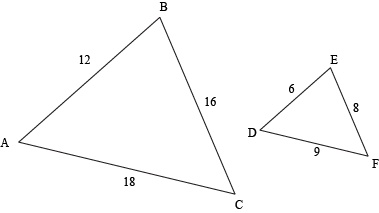ABC is similar to ∆DEF. Mathematicians, always trying to be more efficient and write things with as few characters as possible, would write ∆ABC ~ ∆DEF. Notice how the symbol for similar (~) is pretty close to the symbol for congruent (≅). Think of it like this: being congruent is kind of like being similar, only with a dose of equality mixed in.

One set of ratios we can write compares the sides of ∆ABC with the corresponding sides of ∆DEF.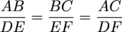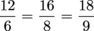We can also write ratios that compare the sides within each triangle. For example,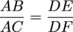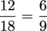• ### Triangle Proportionality Theorem

Similar triangles can be located any number of places, including one inside the other. And if you're working with a big problem, there may be a third similar triangle inside of the first two. And technically there could be a fourth one, even smaller, inside of the third.

But before Leonardo DiCaprio or Joseph Gordon-Levitt show up and draw us into a ridiculous triangle-ception situation, let's just state for the record that we're only going to deal with the first two, which are actually created by taking one triangle and shooting a line through it. Because if that line is parallel to one side of the triangle, it'll create two similar triangles.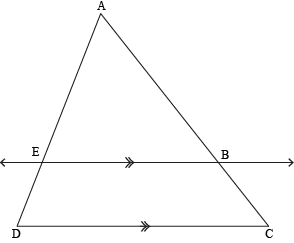Here, ∆ACD ~ ∆ABE. We'll prove this a little later. For now, we're going to focus on the chopped-up side pieces.

In this illustration, line EB is parallel to side DC. We can see that it intersects sides AC and AD. This creates proportional segments: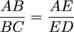In fact, we even have a theorem about this: the Triangle Proportionality Theorem. Apparently, mathematicians got quite a giggle when they first came up with it, since it's earned the nickname "The Side-Splitter Theorem." To be honest, we don't really get it. It must be an inside joke.

The Triangle Proportionality Theorem says that if a line is parallel to one side of a triangle, then it splits the other two sides into proportional sections.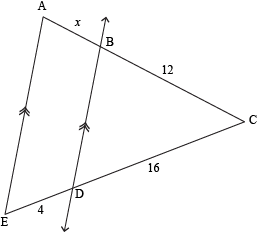We can use this theorem to find the value of x in ∆ACE. We're given that line BD is parallel to side AE, and three of the resulting segment lengths are also given. To find the missing piece, set up a proportion comparing the side lengths:

164 = 12x

Now cross-multiply and solve for x:

16x = 48
x
= 3

Or, the quick way would be to notice that 164 = 4, so 12x must also equal 4, which would also give us x = 3.

### Sample Problem

Find the value of y.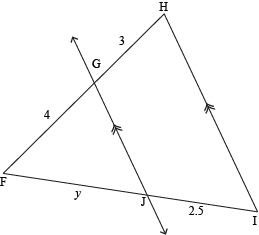We'll solve this one with a proportion. The TPT says we can.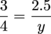Cross-multiply to get 3y = 10. Divide both sides by 3 get y = 3.3.

You may have recognized the TPT as a classic If-Then conditional statement. And this time, the converse is true: if a line splits two sides of a triangle into proportional sections, then the line is parallel to the third side.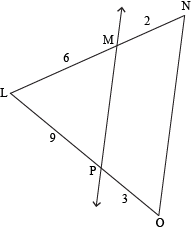Since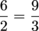, we know that MP || NO.

We're kind of on a roll here with the conditional statements, so let's keep going with the contrapositive of the TPT: if the side segments aren't proportional, then the line that created them isn't parallel to the third side. True?

Of course, true! (If the statement is true, then the contrapositive is also full of truthiness.)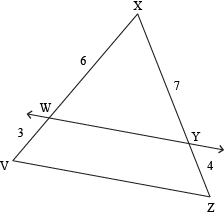Here,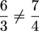, so WY is not parallel to VZ.

### Sample Problem

State whether QS || PT.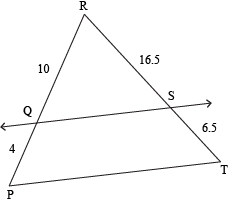If the segment lengths are proportional, then we know the line is parallel to the side. And, if they're not proportional, the line isn't parallel to the side. In this case,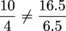, so no, QS is not parallel to PT.

• ### Triangle Midsegment Theorem

The Triangle Midsegment Theorem. TMT. Sounds like fun, yes?

While not nearly as scandalous as TMZ, the TMT shares plenty of juicy morsels of gossip about the lengths of various line segments in and around the world of triangles. Specifically, it says that if you connect the midpoints of two sides of a triangle, then you've got yourself a midsegment, a magical creature that lives smack dab in the middle of the triangle it calls home. Midsegments are half the length of the side they run parallel to, they bisect the other two sides, and they fart glitter. No, wait, that's unicorns.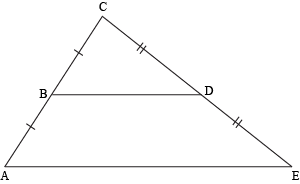Here, B is the midpoint of AC, and D is the midpoint of CE. We can be sure of that because we're told that the segments are congruent on each side of both of those points. Connecting them, we get the midsegment BD. TMT says that BD || AE and that BD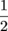× AE.

### Sample Problem

If GI = 11, how long is FJ?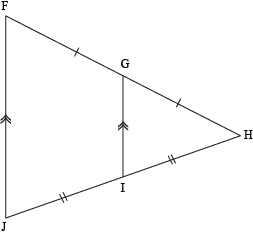Well, we can see that GI is a midsegment, since FGGH, JIIH, and FJ || GI. This means that FJ is twice GI, so FJ = 2 × 11 = 22.

One interesting side note, or corollary if you want to be technical, is that if three parallel lines all intersect two transversals, and they create congruent segments on one of those transversals, then the segments on the other transversal are also congruent. In Triangle Land (wouldn't that be a fun theme park?), this tells us that if we have a line parallel to one side that intersects a second side at its midpoint, then it automatically intersects the third side at its midpoint as well.

(Yes, we did say three parallel lines. The third parallel line goes through the vertex opposite the first side. It's invisible, like your little brother's imaginary friend, Buster the Space Ranger.)So, if we look at ∆FJH again, where we're given that G is the midpoint of FH and FJ || GI, then we can figure out that I is the midpoint of JH. Thanks, TMT.

### Sample Problem

VXY has midsegment WZ, where W is the midpoint of VX. If VX = 14 and VZ = 4, how long are VW and VY?

Because WZ is a midsegment, we know it cuts VX and VY each in half, like this: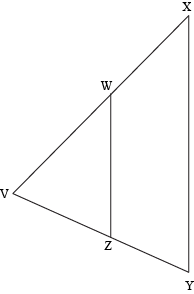Since VX = 14 and we're slicing it in half, we know that VW = 7. And because VZ = 4, VY = 8.

But we can take this one step further. Not only does this corollary work for congruent segments, but for any proportional segments. Yep, more proportions. Please don't hate us.

Parallel lines that intersect transversals create proportional segments on those transversals.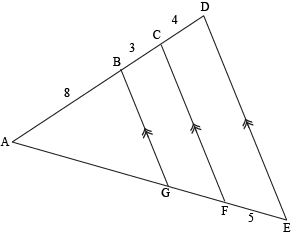In ∆ADE, we can set up the following proportions to find the missing lengths: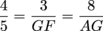. Let's deal with each of these separately.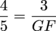Cross-multiply to get 4 × GF = 15. Divide both sides by 4 and we find GF = 3.75.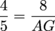Again, cross-multiply and we get 4 × AG = 40. Divide both sides by 4 (whoa, déjà vu) and this time we have AG = 10.

### Sample Problem

Find the values of a and b.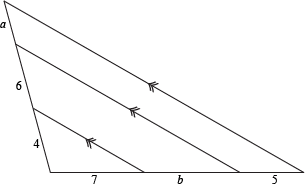Thanks to TMT and its corollaries, we can set up proportions to find the missing lengths.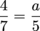7a = 20
a = 2.857142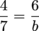4b = 42
b = 10.5

• ### Angles and Sides

Are you tired of hearing that similar triangles have congruent angles and proportional sides? We only keep saying it because it's important. And true. And important.

If we know that two triangles have congruent angles and proportional sides, then we know that they're similar. Actually, if we know that two triangles have congruent angles and/or proportional sides, then we know that they're similar. We don't have to know both, though it is nice when you've got all your bases covered.

Check out these triangles.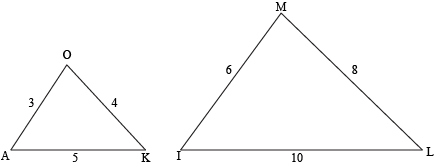They sure do look similar, but sometimes drawings can throw us a curveball. Fortunately, the triangles' side lengths are given to us, which is handy, because we can't tell just from looking whether the angles are congruent.

Let's set up the ratios and compare. Are they equal?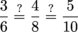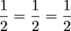Yes, indeed, the side lengths are proportional, so we can be sure that ∆OAK ~ ∆MIL.

### Sample Problem

PIT has side lengths 4, 5, and 8, while ∆KAN has side lengths 12, 15, and 22. Are they similar?

Let's set up some ratios and see.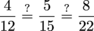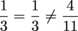Nope. So they're not similar.

It's a whole 'nother ball game when we're dealing with angles. While side lengths are proportional in similar triangles, the angles have to be congruent. If we cut out any two similar triangles (which we wouldn't recommend doing to your screen, but feel free to print the page and then proceed with the paper) and placed them on top of each other, we could line up each set of corresponding angles and see that the angles are exactly the same size.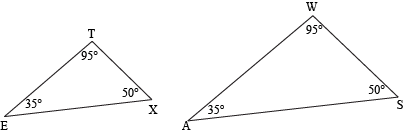Triangles ∆TEX and ∆WAS are similar because their angles are congruent. Specifically, ∠T ≅ ∠W, ∠E ≅ ∠A, and ∠X ≅ ∠S.

### Sample Problem

NYM has angles that measure 50°, 87°, and 43°. ∆PHI has angles that measure 87°, 50°, and 43°. Are they similar?

Yep. Mark it down: a triple play with three pairs of congruent angles. That makes the triangles similar.

This begs an interesting question. Do we have to know the measures of all the angles in order to decide whether two triangles are similar?

In short, no.

The long answer relies on the fact that the three angles inside every triangle always add up to 180° (remember the Angle Sum Theorem for Triangles?). That means that if we only know the measure of two of the angles, we can figure out the measure of the third one. Ballpark figures won't cut it, though.

So what about these two triangles? Are they similar?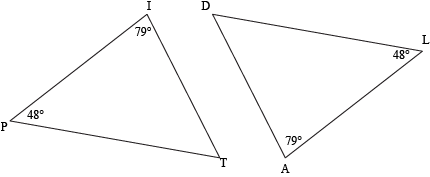In the first triangle, we know ∠P = 48° and ∠I = 79°. Since m∠P + m∠I + m∠T = 180, we can calculate that m∠T = 53°. In the second triangle, m∠L = 48° and m∠A = 79°. Again, since m∠L + m∠A + m∠D = 180, we know that ∠D must be 53° as well. Both triangles have three congruent angles, which means they must be similar.

To write the similarity statement, we have to get our letters in the right order. Congruent angles must be in the same place in each triangle's name: ∠P ≅ ∠L, ∠I ≅ ∠A, and ∠T ≅ ∠D, so we write ∆PIT ~ ∆LAD.

But we really didn't have to calculate the size of those third angles. Here's why:

 Statements Reasons 1. m∠P + m∠I + m∠T = 180 Angle Sum Theorem for Triangles 2. m∠L + m∠A + m∠D = 180 Angle Sum Theorem for Triangles 3. m∠P = m∠L, m∠I = m∠A Given (in figure) 4. m∠T = 180 – (m∠P + m∠I) Rearranging (1) 5. m∠D = 180 – (m∠L + m∠A) Rearranging (2) 6. m∠T = 180 – (m∠L + m∠A) Substitution (3, 4) 7. m∠D = m∠T Substitution (5, 6) 8. ∠D ≅ ∠T Definition of Congruent Angles (7)

Even without needing the exact measurements of any of the angles, we know that ∠T and ∠D are equal in measure, meaning the that they're also congruent.

And we've just discovered something called the Angle-Angle Postulate, which says that if two triangles share two pairs of congruent angles, then the triangles are similar. See, isn't sharing nice?

### Sample Problem

Two of the angles in ∆BOS measure 22° and 108°, while two angles in ∆CLE measure 108° and 48°. Are the triangles similar?

We know from the problem that they have at least one congruent angle (108°). But do they have another set? To find the missing angle in ∆BOS, we'll solve 180° – (108° + 22°) = x, or x = 50°. The three angles in ∆BOS are 22°, 50°, and 108°. None of them is 48°, so we already know (without figuring out the size of ∆CLE's third angle) that our two triangles don't have at least two congruent angles. They don't match, so the two triangles are not similar. A swing and a miss.